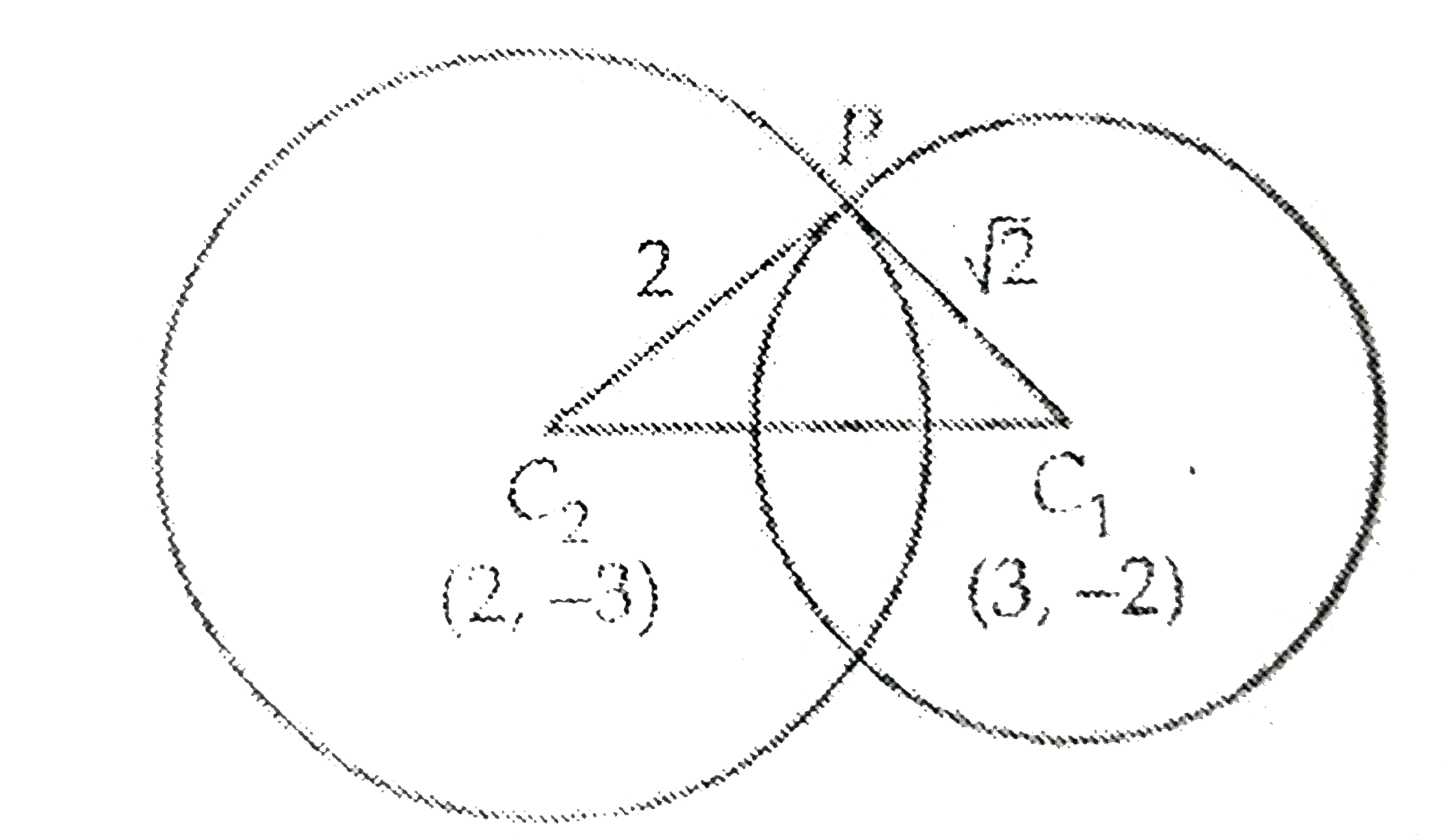Doubtnut App - Get 50 Lakh+ video solutions better than GoogleInstall Now

# Find the angle of intersection of the circles x2+y2−6x+4y+11=0andx2+y2−4x+6y+9=0

A

30

B

45

C

60

D

90

Text Solution
Verified by Experts

## The equations of the circles are x2+y2−6x+4y+11=0 ...(i) x2+y2−4x+6y+9=0 ...(ii) The centres of these circles are C1(3,−2)andC2(2,−3) respectively. Let r1andr2 be their radii. Then, r1=√9+4−11=√2andr2=√4+9−9=2.Also, C1C2 Suppose circles (i) and (ii) intersect at P. Then, cos∠C1PC2=C1P2+C2P2−C1C222C1P.C2P=2+4−22×√2×2=1√2 ⇒∠C1PC2=45∘ Thus, the angle of intersection of the given circles is of 45∘.